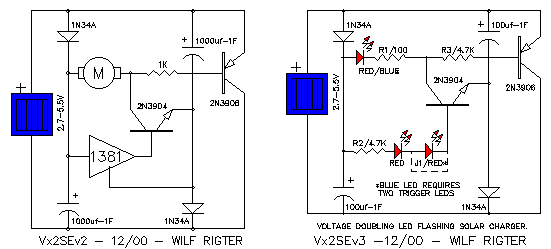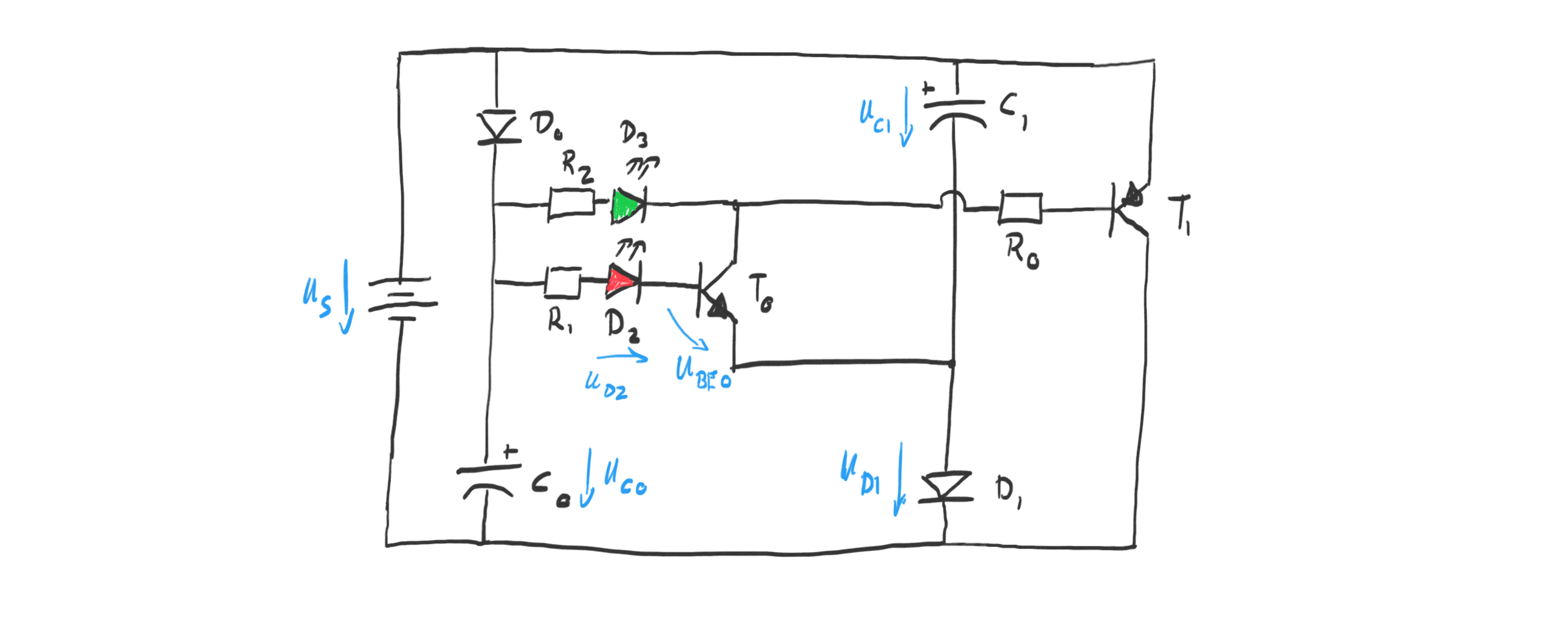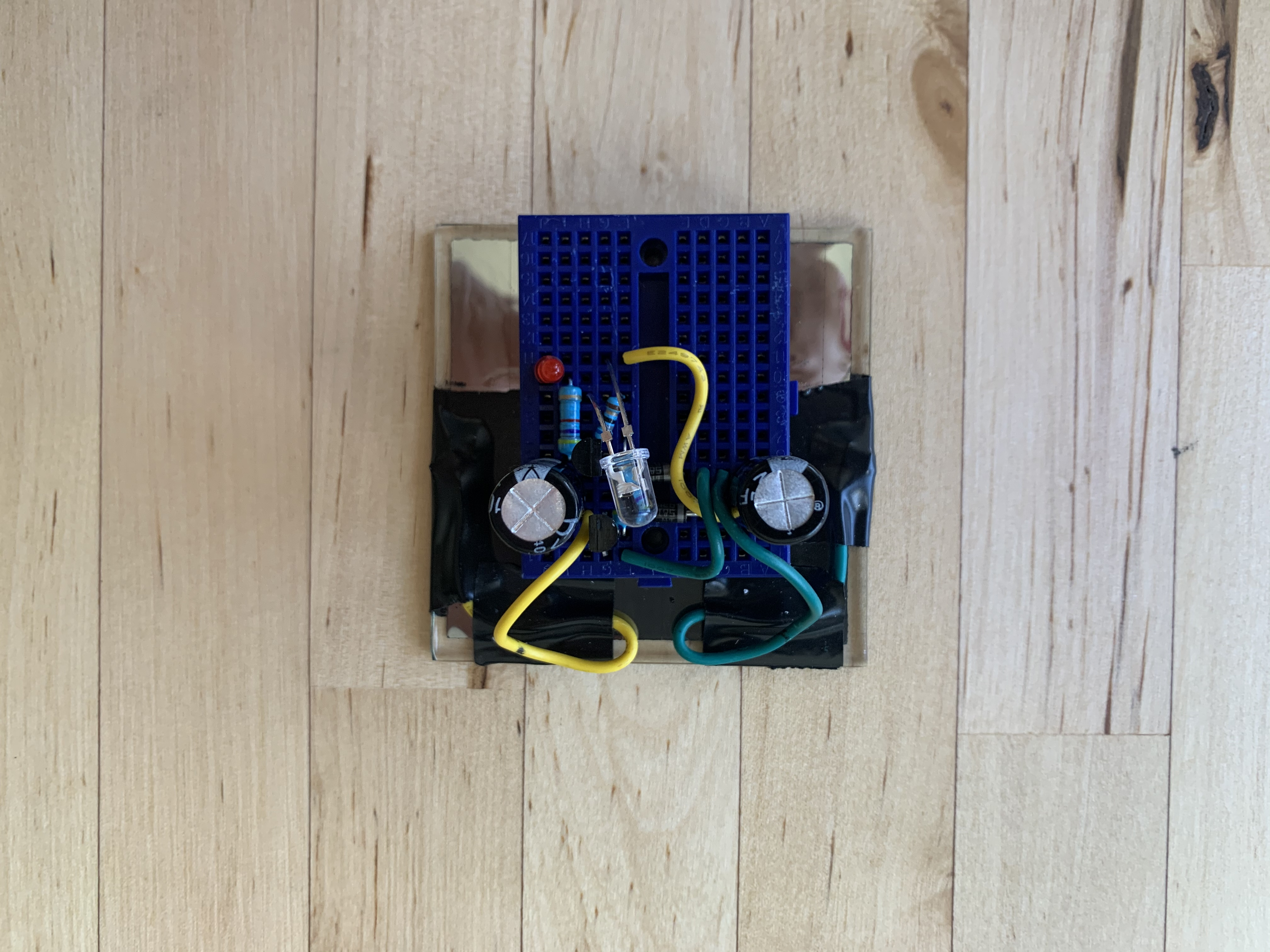# Julian Straub

## creations and thoughts

Building the pretty straight forward light harvester described in a previous post, I learned that that design is somewhat passive: the harvester just charges the whole day and then discharges for 1-2h (depending on the capacitor) once it gets dark.To explore a more active harvester that periodically emits energy whenever the capacitor is charged, I reimplemented the VxSE3 solar engine by WLF Rigter:Reproduced here with my specific adaptations:

• used a green LED with forward voltage of 3.2V
• a solar panel with 3V output (max 4V)
• $$10\mu F$$ storage capacitorsThe circuit above is pretty clear for the charging cycle: the capacitors are charged in parallel each through its own diode. I used Schottky diodes with a forward drop of 0.4V. Given the 3V solar cell this means they will each be charged to about $$U_{c0} = U_{c1} = 2.6V$$ .

Once the capacitors reach their full charge the transistor $$T_0$$ goes into conduction. We can see that by looking at the voltage $$U_{BE0}$$ :

$$U_{BE0} = U_{c0} - U_{d1} - U_{d2} - U_{R1} = 2.6V - 1.8V - U_{R1} = 0.8V - U_{R1}$$

which is $$>0.7V$$ since the resistor just acts to limit the current into the transistor.

With $$T_0$$ saturated and in conduction it is instructive to look at this re-drawing of the circuit to understand the voltage doubling feature of this circuit:As soon as $$T_0$$ is in conduction, the output of the green LED $$D_3$$ is pulled to the potential of the lower capacitor $$C_1$$ . The base of the pnp transistor $$T_1$$ is pulled to the same potential as well. The saturates $$T_1$$ as can be seen from the base-emitter voltage of $$T_1$$ :

$$U_{BE1} = -U_{R0} - U_{c1} + U_{BE0} \approx -2.4V -U_{R0} < -0.7V$$

The saturated npn transistor $$T_1$$ then connects the two capacitors leading to an approximate doubling of the input voltage. More precisely, the following voltage is applied over the green LED and its resistor:

$$U_{D3} + U_{R2} = U_{c0} + U_{c1} - U_{CE1} - U_{CE0} \approx 2 U_{c0} - 0.4V = 4.8V$$

This doubling of the voltage reminds of a charge pump circuit.

The resistor $$R_2$$ limits the discharge current through the green LED $$D_3$$ as usual, in the most simple LED circuit. As the capacitors discharge the voltage eventually drops too low to keep transistor $$T_0$$ in conduction and the circuit resets to charging mode.The initial version used the parts as described in the original engine:

• $$R_0=4.7k\Omega, R_1=4.7k\Omega, R_2=68\Omega, C_0=C_1=1mF$$ .
• $$D_0=D_1=$$ Schottky
• $$T_0=$$ 2N3904 and $$T_1=$$ 2N3906
• $$D_2=$$ low power red LED
• $$D_3=$$ one ultra-bright green LED.
• 4V max - rated for 3V solar cell.